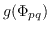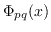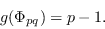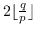## Bin Zhang: Remarks on the maximum gap in binary cyclotomic polynomials, p.109-115

### Abstract:

Let p < q be odd primes, and letdenote the maximum of the differences (gaps) between two consecutive exponents occurring in the pq-th cyclotomic polynomial. In this note, we give an alternative proof of the following result which was established by Hong et al. [J. Number Theory, 132 (2012), pp. 2297-2315]In addition, we show that the number of maximum gaps inis given by.

Key Words: Cyclotomic polynomial, Binary cyclotomic polynomial, Maximum gap.

2000 Mathematics Subject Classification: Primary: 11C08;
Secondary: 11B83..